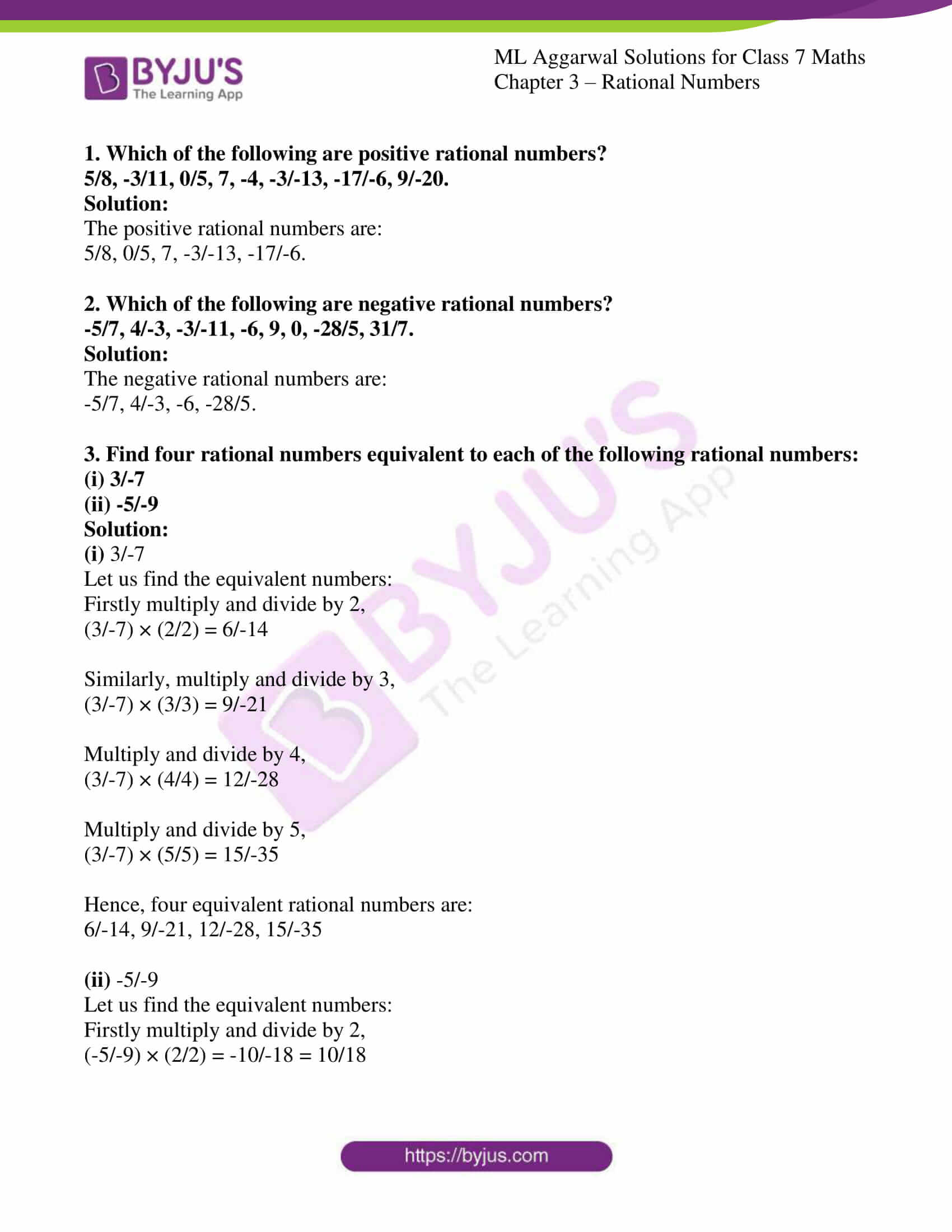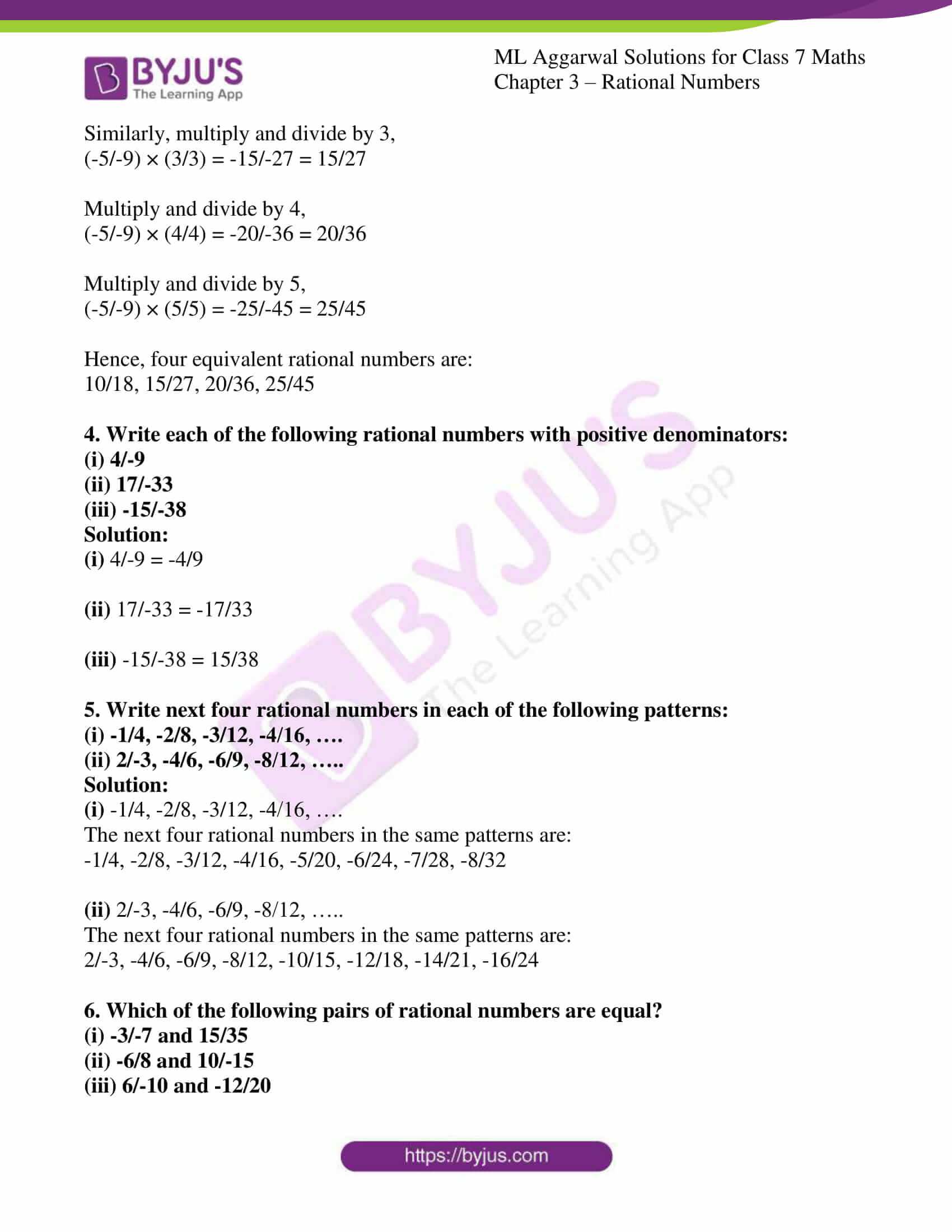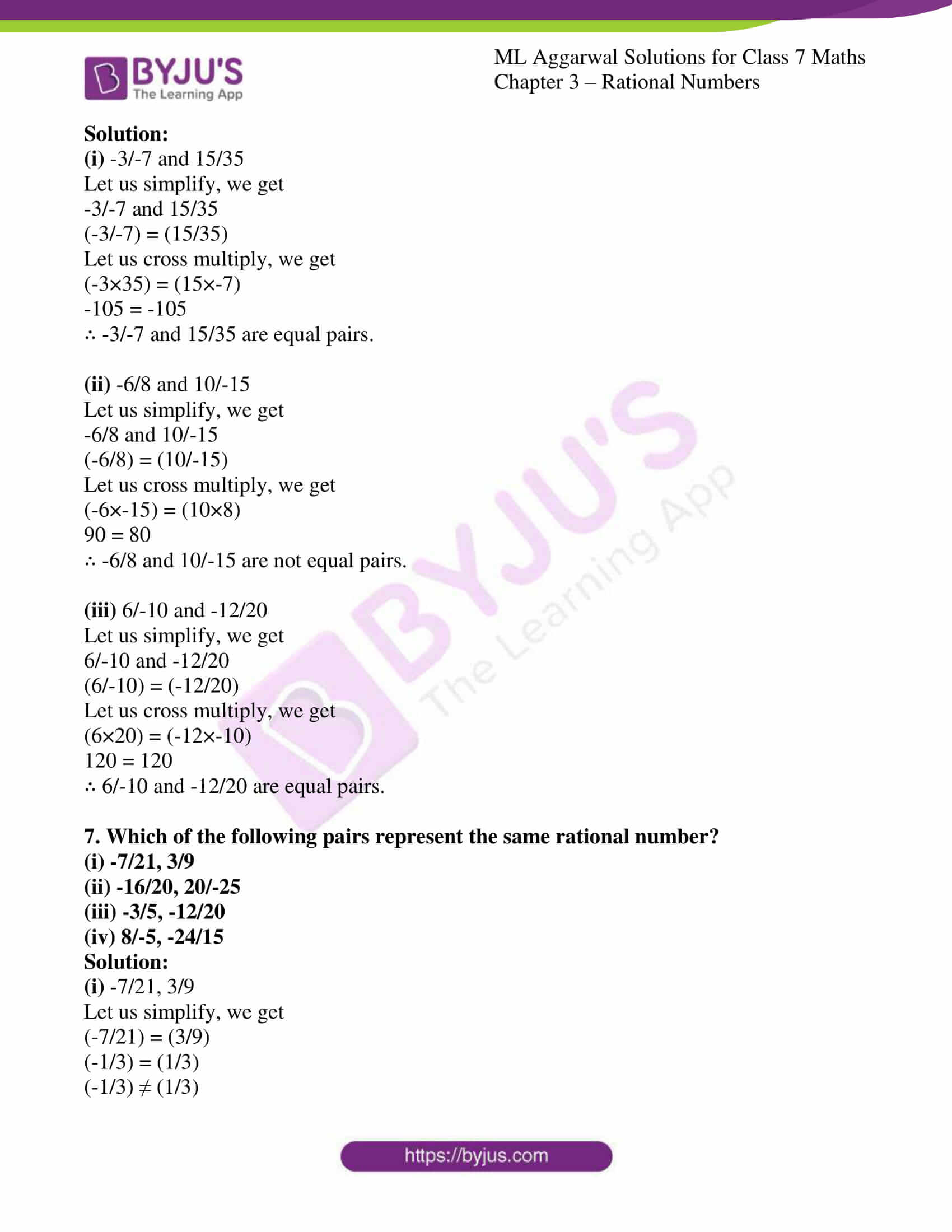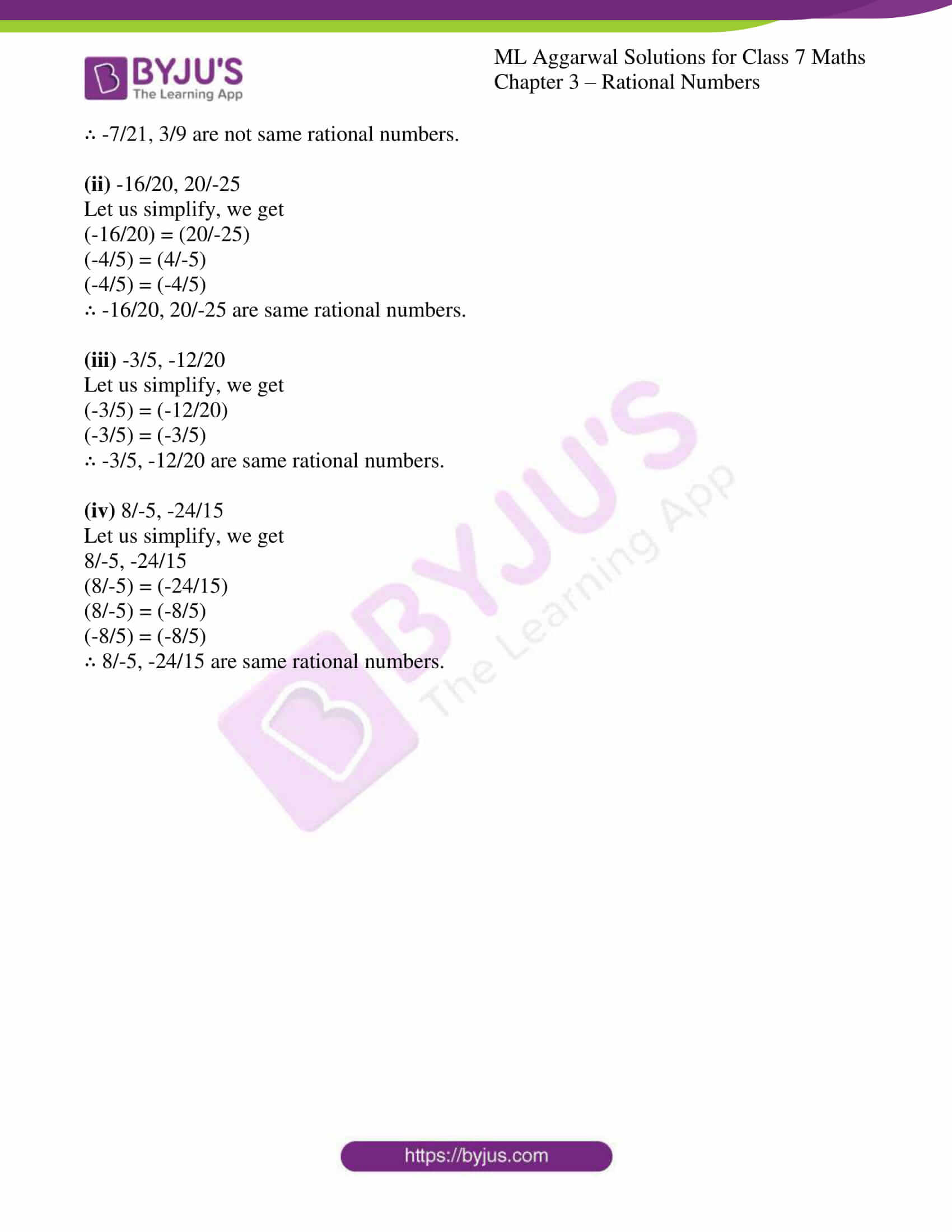# ML Aggarwal Solutions for Class 7 Maths Chapter 3 - Rational Numbers

ML Aggarwal Solutions for Class 7 Maths Chapter 3 – Rational Numbers are provided here to help students prepare for their exams and score well. This chapter mainly deals with problems based on rational numbers. To make learning easy and fun, the solutions here are developed in an interesting manner to help students grasp the concepts easily. With ML Aggarwal Solutions students can effortlessly prepare for their exams and are also advised to practice on a daily basis to excel in the board exams. Students can easily download the solutions and can start practising offline, in order to gain that extra edge of knowledge.

Chapter 3 – Rational Numbers provides solutions to questions related to each and every topic present in this chapter. Students can refer and easily download the pdf of ML Aggarwal Class 7 Solutions for free, from the links given below.

## Download the Pdf of ML Aggarwal Solutions for Class 7 Maths Chapter 3 – Rational Numbers### Access answers to ML Aggarwal Solutions for Class 7 Maths Chapter 3 – Rational Numbers

1. Which of the following are positive rational numbers?

5/8, -3/11, 0/5, 7, -4, -3/-13, -17/-6, 9/-20.

Solution:

The positive rational numbers are:

5/8, 0/5, 7, -3/-13, -17/-6.

2. Which of the following are negative rational numbers?

-5/7, 4/-3, -3/-11, -6, 9, 0, -28/5, 31/7.

Solution:

The negative rational numbers are:

-5/7, 4/-3, -6, -28/5.

3. Find four rational numbers equivalent to each of the following rational numbers:
(i) 3/-7
(ii) -5/-9
Solution:

(i) 3/-7

Let us find the equivalent numbers:

Firstly multiply and divide by 2,

(3/-7) × (2/2) = 6/-14

Similarly, multiply and divide by 3,

(3/-7) × (3/3) = 9/-21

Multiply and divide by 4,

(3/-7) × (4/4) = 12/-28

Multiply and divide by 5,

(3/-7) × (5/5) = 15/-35

Hence, four equivalent rational numbers are:

6/-14, 9/-21, 12/-28, 15/-35

(ii) -5/-9

Let us find the equivalent numbers:

Firstly multiply and divide by 2,

(-5/-9) × (2/2) = -10/-18 = 10/18

Similarly, multiply and divide by 3,

(-5/-9) × (3/3) = -15/-27 = 15/27

Multiply and divide by 4,

(-5/-9) × (4/4) = -20/-36 = 20/36

Multiply and divide by 5,

(-5/-9) × (5/5) = -25/-45 = 25/45

Hence, four equivalent rational numbers are:

10/18, 15/27, 20/36, 25/45

4. Write each of the following rational numbers with positive denominators:
(i) 4/-9
(ii) 17/-33
(iii) -15/-38
Solution:

(i) 4/-9 = -4/9

(ii) 17/-33 = -17/33

(iii) -15/-38 = 15/38

5. Write next four rational numbers in each of the following patterns:

(i) -1/4, -2/8, -3/12, -4/16, ….

(ii) 2/-3, -4/6, -6/9, -8/12, …..

Solution:

(i) -1/4, -2/8, -3/12, -4/16, ….

The next four rational numbers in the same patterns are:

-1/4, -2/8, -3/12, -4/16, -5/20, -6/24, -7/28, -8/32

(ii) 2/-3, -4/6, -6/9, -8/12, …..

The next four rational numbers in the same patterns are:

2/-3, -4/6, -6/9, -8/12, -10/15, -12/18, -14/21, -16/24

6. Which of the following pairs of rational numbers are equal?

(i) -3/-7 and 15/35

(ii) -6/8 and 10/-15

(iii) 6/-10 and -12/20

Solution:

(i) -3/-7 and 15/35

Let us simplify, we get

-3/-7 and 15/35

(-3/-7) = (15/35)

Let us cross multiply, we get

(-3×35) = (15×-7)

-105 = -105

∴ -3/-7 and 15/35 are equal pairs.

(ii) -6/8 and 10/-15

Let us simplify, we get

-6/8 and 10/-15

(-6/8) = (10/-15)

Let us cross multiply, we get

(-6×-15) = (10×8)

90 = 80

∴ -6/8 and 10/-15 are not equal pairs.

(iii) 6/-10 and -12/20

Let us simplify, we get

6/-10 and -12/20

(6/-10) = (-12/20)

Let us cross multiply, we get

(6×20) = (-12×-10)

120 = 120

∴ 6/-10 and -12/20 are equal pairs.

7. Which of the following pairs represent the same rational number?

(i) -7/21, 3/9

(ii) -16/20, 20/-25

(iii) -3/5, -12/20

(iv) 8/-5, -24/15

Solution:

(i) -7/21, 3/9

Let us simplify, we get

(-7/21) = (3/9)

(-1/3) = (1/3)

(-1/3) ≠ (1/3)

∴ -7/21, 3/9 are not same rational numbers.

(ii) -16/20, 20/-25

Let us simplify, we get

(-16/20) = (20/-25)

(-4/5) = (4/-5)

(-4/5) = (-4/5)

∴ -16/20, 20/-25 are same rational numbers.

(iii) -3/5, -12/20

Let us simplify, we get

(-3/5) = (-12/20)

(-3/5) = (-3/5)

∴ -3/5, -12/20 are same rational numbers.

(iv) 8/-5, -24/15

Let us simplify, we get

8/-5, -24/15

(8/-5) = (-24/15)

(8/-5) = (-8/5)

(-8/5) = (-8/5)

∴ 8/-5, -24/15 are same rational numbers.